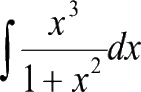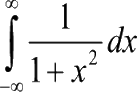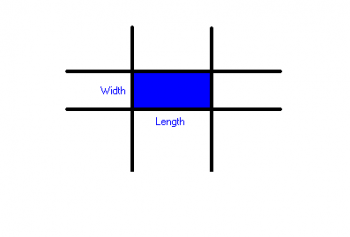Games
Problems
Go Pro!

Problems > Mr. T's Calculus > 2002

## A challenging antiderivative

Find the following antiderivative:## What's the Value (or does it diverge?)

Find the value of the following integral, or show that it diverges.## Piecewise defined FUNctions

Given the function defined by:
f(x) = x2 + bx, if x<2
f(x) = bx + c, if x ≥ 2

Determine the ordered triple (a, b, c) so that all of the following conditions are true:

1. f (x) is continuous
2. f (3) = 20
3.
lim f(x)
x→2
=0

## What's my equation?

The graph shown below consists of a line segment connected to a piece of a parabola, with the intersection at (0, 2). The derivative at the point of intersection is continuous, and each tick mark on the axes represents one unit. Find the equation of the parabola in the form p(x) = ax2 + bx + c.## How fast does it change?

A particular dance is performed with four moving poles. At any one instant in time, these poles form a rectangle. The poles forming each pair of opposite sides move closer together and farther apart throughout the course of the dance. The dancer is to step into this rectangle and out again in such a way that he or she never gets pinched by the poles. In the diagram shown, assume that the Length of the rectangle varies according to the equation L = 4 + 3cos t, and the Width of the rectangle varies according to the equation W = 3 + 3sin t, where t is in seconds, and the dimensions are in feet. The area, A, then varies as a function of time.Find the rate at which the area is changing at time t = 5 seconds. Be sure to indicate whether the area is increasing or decreasing.

## What's my distance?

There is more than one way to solve this particular problem. Please feel free to suggest an alternate solution, IN ADDITION to the method I have requested. If you provide an appropriate alternate solution, I may be inclined to give additional bonus points!

Please provide an explanation other than "I put the data in my calculator and ran a program."

The velocity from a speedometer on a car was recorded over a one-hour time span. The results appear in the table below.

Time (min)
Velocity (mph)
0
25
5
30
15
50
20
40
25
45
30
25
40
30
45
45
50
30
55
40
60
50

Compute an approximation to the distance traveled by computing an Upper Riemann Sum, a Lower Riemann Sum, and then finding the mean of those two values. Please give the values of the two computed sums, in addition to your final answer, as part of your solution.Like us on Facebook to get updates about new resources